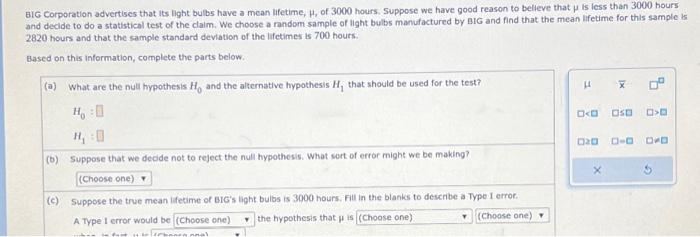# (Solved): BIG Corporation advertises that its light bulbs have a mean lifetime, , of 3000 hours. Suppose we ...BIG Corporation advertises that its light bulbs have a mean lifetime, , of 3000 hours. Suppose we have good reason to belleve that is less than 3000 hours and decide to do a statistical test of the claim. We choose a mndom sample of Hght bulbs manufactured by BIG and find that the mean lifetime for this sample is 2820 hours and that the sample standard deviation of the lifetimes is 700 hours. Based on this information, complete the parts below. (a) What are the null hypothesis and the alternative hypothesis that should be used for the test? (b) Suppose that we dedde not to reject the null hypothesis. What sort of error might we be making? (c) Suppose the true mean ifeame of sic's light bulbs is 3000 hours. Fill in the blanks to describe a Type I erroc. A Type 1 error would be the hypothesis that is

We have an Answer from Expert